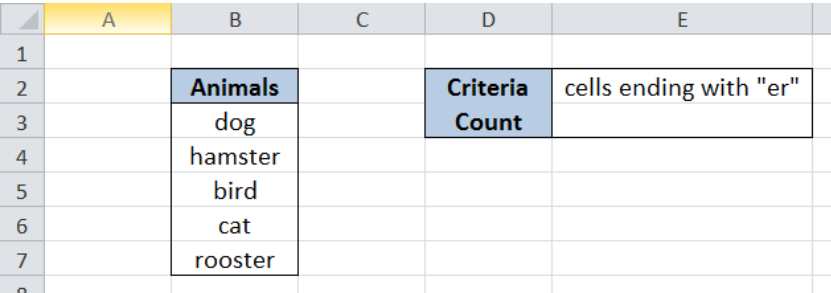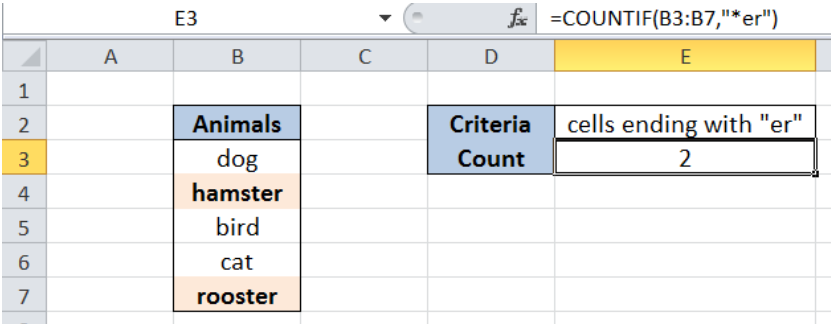Get instant live expert help with Excel or Google Sheets“My Excelchat expert helped me in less than 20 minutes, saving me what would have been 5 hours of work!”

#### Post your problem and you’ll get Expert help in seconds.

Your message must be at least 40 characters
Our professional Expert are available now. Your privacy is guaranteed.

# Count cells that end with

While working with Excel, we are able to count values in a data set based on a given criteria by using the COUNTIF function.  COUNTIF returns the number of values in a specified range based on a given condition.  This step by step tutorial will assist all levels of Excel users in counting values that end with a specific text or character.Figure 1. Final result: Count cells that end with “er”

Final formula:  `=COUNTIF(B3:B7,"*er")`

## Syntax of COUNTIF Function

`=COUNTIF(range,criteria)`

Parameters:

• range – the data range that will be evaluated using the criteria
• criteria – the criteria or condition that determines which cells will be counted

## Setting up Our Data

Our table contains a list of Animals (column B). Cell E2 contains the criteria that we want to apply which is cells ending with “er”.  In cell E3, the count based on the given criteria will be recorded.Figure 2. Sample data to count cells that end with “er”

## Count cells that end with “er”

In order to determine the number of cells that end with “er”, we follow these steps:

Step 1.  Select cell E3

Step 2.  Enter the formula: `=``COUNTIF(B3:B7,"*er")`

Step 3: Press ENTERFigure 3. Using COUNTIF and asterisk “*” to count cells that end with “er”

Our range for the COUNTIF function is the range B3:B7 which contains our data for “Animals”.  The criteria is “*er” which means that we want to count the animals whose names end with “er”.  The asterisk “*” is a wildcard in Excel and it means that there could be any number of characters before “er”.

As a result, our formula returns 2, which corresponds to two values that end with “er”: hamster and rooster.

Most of the time, the problem you will need to solve will be more complex than a simple application of a formula or function. If you want to save hours of research and frustration, try our live Excelchat service! Our Excel Experts are available 24/7 to answer any Excel question you may have. We guarantee a connection within 30 seconds and a customized solution within 20 minutes.

### Did this post not answer your question? Get a solution from connecting with the expert.Another blog reader asked this question today on Excelchat:
Solution examplesI need a formula to count the number of rows that contain at least one of two possible phrases, but not give me the total number of instances that both phrases occur overall.
Solved by E. B. in 33 minsi have a table with Dates, First names , Last names and i want to count how many times a name occurs, but if a name occurs more than once on a particular date i need to only count it once. this is on excel and not google sheets
Solved by F. H. in 31 minsNeed a formula that counts (1,2,3,4 ect..in cell M21) a range of cells, N4:N20, which are annual premium values. But I only want to add a sale count if the average monthly premium is above \$30 per sale. So if someone has 4 sales but they don't equal a total of \$120/mth (\$30 x 4 = \$120)... they would only get a count of 3 sales.
Solved by S. E. in 60 minsHello, I am trying to create a total color column? Can anyone help?
Solved by I. A. in 60 minsAny rep that has orders over 500 and occurrences at zero for each month, for a 6 month period. I need a sum
Solved by C. E. in 60 mins## Subscribe to Excelchat.coAnother blog reader asked this question today on Excelchat: